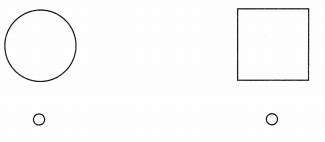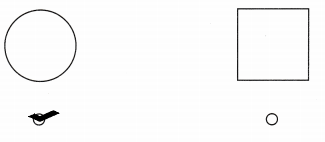# Texas Go Math Kindergarten Lesson 18.5 Answer Key Components of Three-Dimensional Objects

Refer to our Texas Go Math Kindergarten Answer Key Pdf to score good marks in the exams. Test yourself by practicing the problems from Texas Go Math Kindergarten Lesson 18.5 Answer Key Components of Three-Dimensional Objects.

## Texas Go Math Kindergarten Lesson 18.5 Answer Key Components of Three-Dimensional Objects

Essential Question
How can you find two-dimensional shapes on a three-dimensional shape?

Unlock the Problem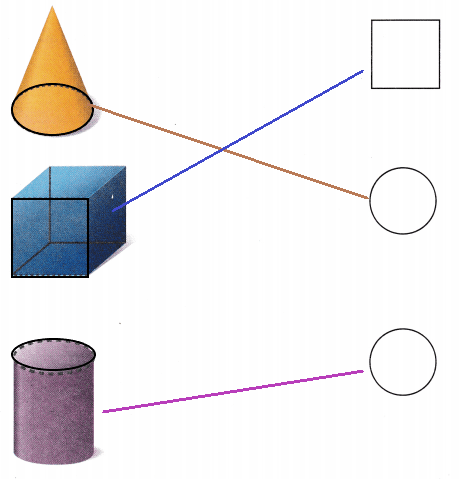Explanation:
I traced the flat surfaces of the given three-dimensional shapes
I matched the shapes with their two-dimensional shapes.

Directions
Trace the dashed part of each three-dimensional shape. Draw lines to match the flat surfaces of the three-dimensional shapes to the two-dimensional shapes.

Try Another Problem

Question 1.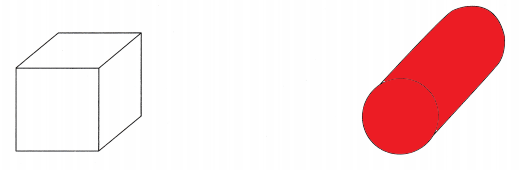Explanation:
A cylinder has a flat surface shaped like a circle
So, i colored the cylinder.

Question 2.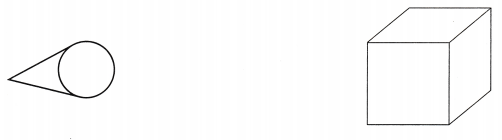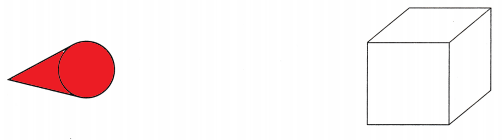Explanation:
A cone does not have a flat shaped like a square
So, i colored a cone.

Question 3.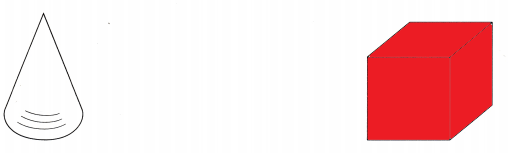Explanation:
A cube has a flat surface shaped like a square
So, i colored a cube.

Directions
1. Which three-dimensional shape has a flat surface shaped like a circle? Color the shape. 2. Which three-dimensional shape does not have a flat surface shaped like a square? Color the shape. 3. Which three-dimensional shape has a flat surface shaped like square? Color the shape.

Share and Show

Question 4.Explanation:
I marked X under the square as the flat surface of the given object is a square.

Question 5.Explanation:
I marked X under the circle as the flat surface of the given object is a circle.

Question 6.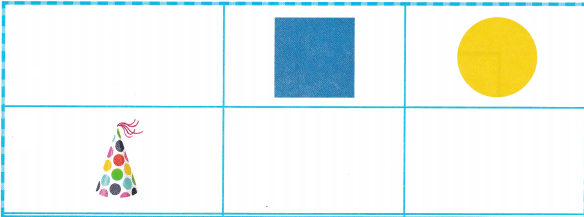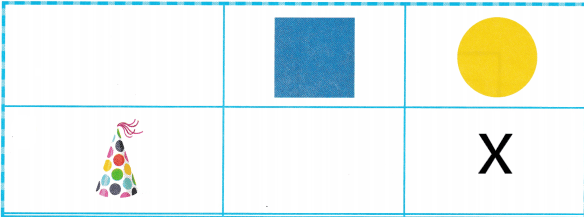Explanation:
I marked X under the circle as the flat surface of the given object is a circle.

Question 7.Explanation:
I marked X under the square as the flat surface of the given object is a square.

Question 8.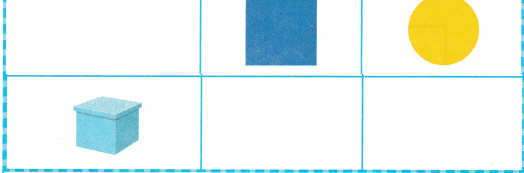Explanation:
I marked X under the square as the flat surface of the given object is a square.

Directions
4-8. Look at the object in the chart. Mark an X under the circle if the flat surface of the object is a circle. Mark on X under the square if the flat surface of the object is a square.

Home Activity

• Have your child identify a household object that is shaped like a three-dimensional shape. Have your child tell you what shape the flat surface of the object is.

Question 9.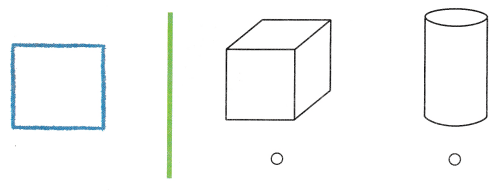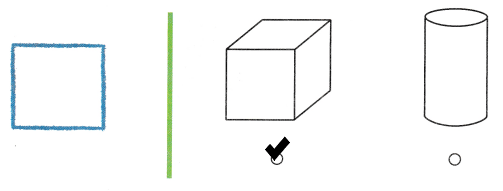Explanation:
Saya traced a block to make a square
Saya used cube as it has a flat surface of square
So, I checked a cube.

Question 10.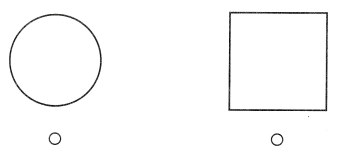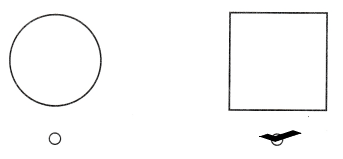Explanation:
I checked a square as it is not a flat surface of a cone.

Question 11.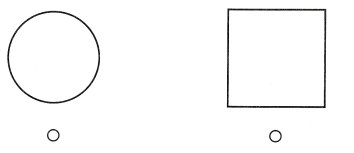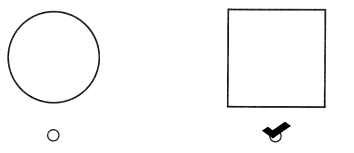Explanation:
I checked square as it is flat surface of a cube.

Directions
Choose the correct answer. 9. Saya traced a block to make a square. Which block did she use? 10. Which shape is not the flat surface on a cone? 11. Which shape is the flat surface on a cube?

### Texas Go Math Kindergarten Lesson 18.5 Homework and Practice

Question 1.Explanation:
I marked X under the circle as the flat surface of the given object is a circle.

Question 2.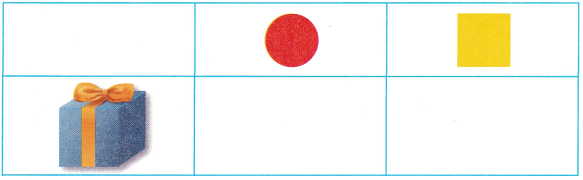Explanation:
I marked X under the square as the flat surface of the given object is a square.

Question 3.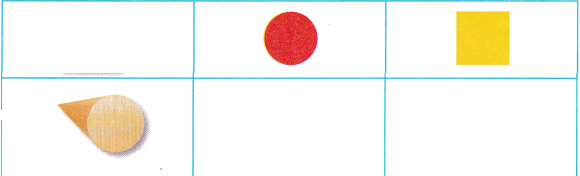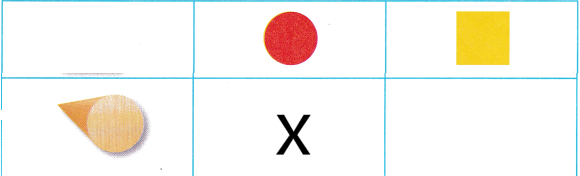Explanation:
I marked X under the circle as the flat surface of the given object is a circle.

Question 4.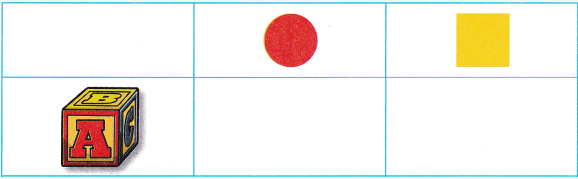Explanation:
I marked X under the square as the flat surface of the given object is a square.

Question 5.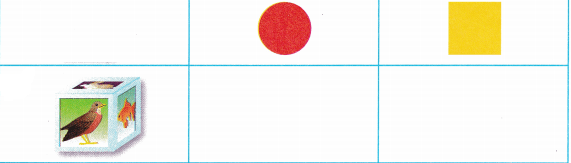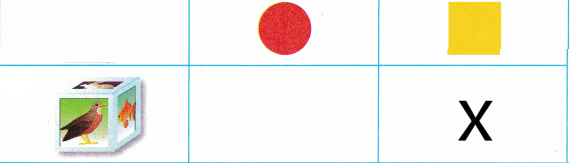Explanation:
I marked X under the square as the flat surface of the given object is a square.

Directions
1-5. Look at the object in the chart. Mark on X under the circle if the flat surface of the object is a circle. Mark on X under the square if the flat surface of the object is a square.

Texas Test Prep

Lesson Check

Question 6.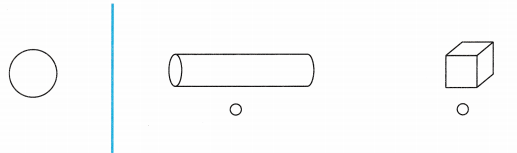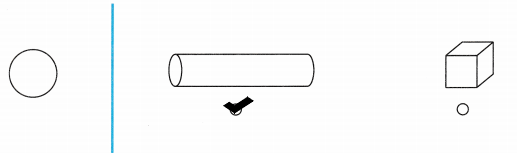Explanation:
I checked a cylinder as Diana traced a cylinder to make a circle.

Question 7.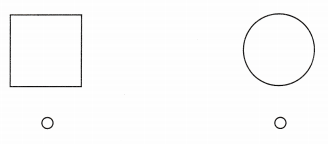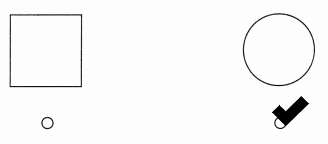Explanation:
I checked the circle as it is the flat surface of a cone.

Question 8.